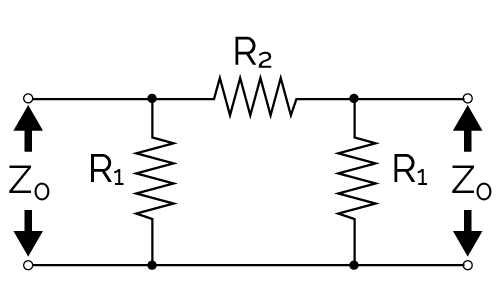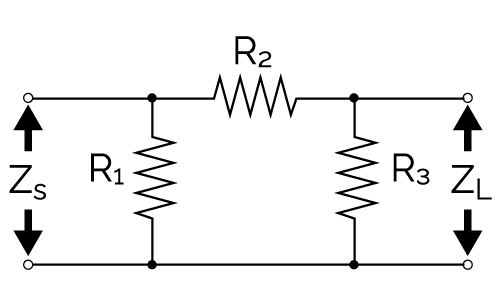# Pi Attenuator Calculator

Created by Luis Hoyos
Reviewed by Hanna Pamuła, PhD and Steven Wooding
Last updated: Feb 02, 2023

With the pi attenuator calculator, you will save the valuable time required in calculating pi attenuator resistor values. You can do it with a couple of slightly complicated formulas or using our pi pad calculator 😎.

Attenuators play valuable functions in electronic circuits, and to achieve those functions, you should calculate the required value of each resistor.

Keep reading this article if you want to know what is a pi attenuator and how to calculate its resistors (using the pi attenuator formula or our pi attenuator calculator). We'll also explore a practical example of a 40 dB attenuator circuit.

## Attenuator basics and the pi attenuator formula

In a broad sense, attenuators are electronic devices that reduce the power of a signal through an amplitude reduction caused by some resistors. Attenuators connect to a source on one side and a load on the other.

Although attenuators are relatively simple devices, we can use them to cause a voltage drop, power dissipation, or improve impedance matching. The resistors inside are the essential components and the architects of these tasks, working as energy dissipators.

Within the family of attenuators, we have the pi attenuator, also known as the "pi circuit" or "pi pad." It's called this way because its topology resembles the Greek "Π." There are two kinds of attenuators within this family:

1. Pi attenuator with equal impedances.
2. Pi attenuator with unequal impedances.

### Pi attenuator with equal impedancesThe equal impedances attenuator is the simplest pi attenuator, as R₁ and R₃ are in parallel and have the same value. Therefore, the source and load impedances are the same.

With the following formula, you can check the results of our calculator:

$\quad\ \ R_1=Z_0\left(\frac{K+1}{K-1}\right)$
$\quad\ \ R_2=Z_0\left(\frac{K^2-1}{2K}\right)$
$\quad\ \ K=10^{\frac{\text{atten}}{20}}$

$R_1$ and $R_2$ are the resulting resistance values, $Z_0$ represents the impedance required, $K$ is called the impedance factor, and $\text{atten}$ is the desired attenuation.

### Pi attenuator with unequal impedancesAre you looking for impedance matching? In that case, the version with unequal impedances is what you need. In this variation, the three resistors have different values, so the input and output impedances differ.

The formula to calculate resistor values is as follows:

$R_1=Z_S\left(\frac{K^2-1}{K^2-2K\sqrt{\frac{Z_S}{Z_L}}+1}\right)$
$R_2=0.5\sqrt{Z_sZ_L}\left(\frac{K^2-1}{K}\right)$
$R_3=Z_L\left(\frac{K^2-1}{K^2-\frac{2K}{\sqrt{Z_S/Z_L}}+1}\right)$

where $R_S$ is the source impedance, $R_L$ is the load impedance, and $R_3$ stands for the third resistor resistance.

## How to use this pi attenuator calculator?

Although the formula to calculate resistances can seem somewhat complicated, our tool is the opposite. This calculator is so straightforward to use that you only need to type the input parameters to get your results in a flash.

For example, if you want to calculate an equal impedances 40 dB attenuator connected to a 50 Ω source and load, these are the steps:

1. Select "Equal impedances" in the "Circuit type" box.
2. Set the attenuation to 40 dB in the second box of the calculator.
3. In the Z₀ box, type a value of 50 Ω.

And that's all! With the previous inputs, your pi attenuator resistor values should be 51 and 2500 Ω for R₁ and R₂, respectively.

Now, if we wanted to solve an unequal impedances 40 dB attenuator circuit connected to a 75 Ω source and a 50 Ω load, these would be the steps:

1. Select "Unequal impedances" in the "Circuit type" box.
2. Set the attenuation to 40 dB in the second box of the calculator.
3. In the ZS box, type a value of 75 Ω.
4. In the ZL box, type 50 Ω.

Now you're done with your resistors! The values should be R₁ = 76.9 Ω, R₂ = 3,062 Ω, and R₃ = 50.8 Ω.

## Using the pi attenuator formula to calculate a 40 dB attenuator circuit

We know confidence in a relationship takes time to build up. So, if you still don't trust our pi pad calculator or us, you can still use the attenuator formula and start to strengthen this bond (or use it to double-check the calculator results).

Let's use again the example in which we wanted to calculate an equal impedances 40 dB attenuator circuit. If we input the same proposed values ($\text{atten}$ = 40 dB and $Z_0$ = 50 Ω), the results are:

$\scriptsize\ \ \ \ K=10^{\frac{\text{atten}}{20}}=10^{\frac{40\ \text{dB}}{20}}=100$
$\scriptsize \ \ \ R_1=Z_0\left(\frac{K+1}{K-1}\right)=50\ Ω\left(\frac{100+1}{100-1}\right)$
$\scriptsize\qquad =50\ Ω\left(\frac{101}{99}\right)=51\ Ω$
$\scriptsize\ \ \ R_2=Z_0\left(\frac{K^2-1}{2K}\right)=50\ Ω\left(\frac{100^2-1}{2\times100}\right)$
$\scriptsize\qquad =50\ Ω\left(\frac{9999}{200}\right)$
$\scriptsize\qquad =2500\ Ω$

The same pi attenuator resistor values we obtained with the pi circuit calculator.

## Applications of pi attenuators

The following are some specific applications of these devices:

• Signal generation: Under working conditions, the source of a signal is usually encountered "naturally" in the circuit in which we're working. But for test purposes, flexible signal sources are a way to study a system's behavior under different possible conditions. Signal generators can achieve this by modifying amplitude, frequency, and wave shape. Attenuators are a tool that can accomplish the task of amplitude (and therefore power) modification.

• Impedance matching: With the aim of minimizing signal reflection or maximizing power transmission in electronics, source and load impedances must be equal (match). When these impedances don't match, you can place an attenuator between them, whose impedance has to match the impedance of the source. In this way, power transmission reaches its maximum value. Unequal impedance attenuators are essential in this case.

• Isolation between circuit stages: Some devices can have problems if directly connected to one another. For example, some amplifiers oscillate if their output directly drives a sharp frequency response ﬁlter. You can use attenuators to provide isolation between these devices.

We're conscious that circuit design is a complex process in which other devices intervene. For that reason, we have designed other calculators beyond the pi circuit calculator that can be very useful to you in this process:

• Impedance matching calculator: Use this tool to know the impedance required in your radiofrequency application. Then, come back here and use it in our pi attenuator calculator with the known impedances.

• VSWR calculator: Isolation between circuit stages (mentioned above) is a way to minimize the voltage standing wave ratio (VSWR) and, in this way, improve impedance matching. After using our pi pad calculator and installing your attenuator, you can use our VSWR calculator to know how your VSWR has improved.

• Cable impedance calculator: To check if there's impedance matching, you first have to know the impedance of the elements, one of them usually a transmission line (i.e., a cable). Remember that the cable impedance calculator requires calculating the wire size and knowing the insulator material.

Luis HoyosCircuit type
Equal impedances
Attenuation
dB
Impedance (Z₀)
Ω
Resistor 1 (R₁)
Ω
Resistor 2 (R₂)
Ω
People also viewed…

### Korean age

If you're wondering what would your age be from a Korean perspective, use this Korean age calculator to find out.

### RAID

This RAID calculator will help you decide which configuration of disks (RAID level) is right for you, based on usable capacity, fault-tolerance, performance, and cost.

### Screen ratio

Our screen ratio calculator allows you to quickly resize your files to fit in a designated area.

### Social Media Time Alternatives

Check what you could have accomplished if you get out of your social media bubble.